# University of Washington, CSE 142

## Lab 3: Parameters, Graphics

Except where otherwise noted, the contents of this document are Copyright 2013 Stuart Reges and Marty Stepp.

lab document created by Marty Stepp, Stuart Reges and Whitaker Brand

# Basic lab instructions

• Mouse over if you're not sure what they mean!
• Talk to your classmates for help.
• You may want to bring your textbook to future labs to look up syntax and examples.
• Stuck? Confused? Have a question? Ask a TA for help, or look at the book or past lecture slides.
• Complete as much of the lab as you can within the allotted time. You don't need to keep working on these exercises after you leave.
• Feel free to complete problems in any order.
• Make sure you've signed in on the sign-in sheet before you leave!

# Today's lab

Goals for today:

• gain a better understanding of how scope works
• learn the basics of declaring and passing parameters to methods
• learn to set a breakpoint with the jGRASP debugger to find out what is going on inside a program
• use the instructor-provided `DrawingPanel` and Java's `Graphics` and `Color` classes

# Scope

Scope: a variable's scope is the part of a program in which it exists. In Java, the scope of a variable starts where it is declared and ends when the closing curly brace for the block that contains it is reached.
 ``` 1 2 3 4 5 6 7 8 ``` ```public static void main(String[] args) { int x = 5; for (int i = 1; i <= 5; i++) { int y = 10; System.out.println(x) // x is still in scope here! } System.out.println(x) // x is still in scope here, too! }```
• `x` is in scope between its declaration on `line 2`, and the curly brace that encloses it on `line 8`.
• `y` is in scope between its declaration on `line 4` and the curly brace that encloses it on `line 6`.
• Loop variables are in scope between their `for` loop's { }. So, `i` is in scope between `lines 3 - 6`.

Note: Two variables with the same name cannot both exist within the same scope.

# Exercise : Scoping 1

What does the following code print out?
 ```1 2 3 4 5 6 7 8 ``` ```public static void main(String[] args) { mystery(); } public static void mystery() { int x = 50; System.out.println(x); }``` Output `50`

# Exercise : Scoping 2

What does the following code print out?
 ```1 2 3 4 5 6 7 8 9 ``` ```public static void main(String[] args) { int x = 15; mystery(); } public static void mystery() { int x = 50; System.out.println(x); }``` Output `50`

# Exercise : Scoping 3

What does the following code print out?
 ``` 1 2 3 4 5 6 7 8 9 10 11 ``` ```public static void main(String[] args) { int x = 15; mystery(); System.out.println(x); } public static void mystery() { int x = 50; x = x + 5; System.out.println(x); }``` Output ```55 15```

# Exercise : Variable Scope

 ``` 1 2 3 4 5 6 7 8 9 10 11 12 13 14 15 16 17``` ```public class Example { public static void main(String[] args) { performTest(); } public static void performTest() { int count = 12; for (int i = 1; i <= 12; i++) { runSample(); System.out.print(count); } } public static void runSample() { System.out.print("sample"); } } ``` In which of these two blocks is the variable `count` in scope? `main` method `for` loop `performTest` method `runSample` method

# Parameters

A parameter allows you to pass in a value (an expression or a variable!) to a method as you call it.

Example:

 ``` 1 2 3 4 5 6 7 8 9 10 11 12 13``` ```public static void main(String[] args) { squared(3); // 3 times 3 is 9 squared(8); // 8 times 8 is 64 int x = 5; squared(x); // 5 times 5 is 25 squared(2 + 2) // 4 times 4 is 16 } public static void squared(int num) { System.out.println(num + " times " + num + " is " + (num * num)); }```

# Exercise : Parameters 1

What does the following code print out?
 ``` 1 2 3 4 5 6 7 8 ``` ```public static void main(String[] args) { int x = 15; mystery(x); } public static void mystery(int y) { System.out.println(y); }``` Output `15`

# Exercise : Parameters 2

What does the following code print out?
 ``` 1 2 3 4 5 6 7 8 9 ``` ```public static void main(String[] args) { int x = 15; int y = 20; mystery(x); } public static void mystery(int y) { System.out.println(y); }``` Output `15`

# Value Semantics

When passing around `int`s, `double`s, `char`s, or `String`s, we need to consider value semantics.

Recall this example:
 ```1 2 3 4 5``` ```public static void main(String[] args) { int x = 5; int y = x; x = 10; // note that y still = 5. It does not change values as x changes. }```

continued on next slide...

# Value Semantics (continued)

This concept persists within parameters. For example:

 ```1 2 3 4 5 6 7 8 9 ``` ```public static void main(String[] args) { int x = 5; mystery(x); // x still equals 5. } public static void mystery(int x) { x = x + 5; // the value of x in mystery is now 10. main's x is unchanged. }```

# Exercise : Parameters 3

What does the following code print out?
 ``` 1 2 3 4 5 6 7 8 9 10 ``` ```public static void main(String[] args) { int x = 15; mystery(x); System.out.println(x); } public static void mystery(int x) { x = x + 50; System.out.println(x); }``` Output ```65 15```

# Parameter Ordering

When passing multiple parameters, order matters. For example:
 ```1 2 3 4 5 6 7 8 9 ``` ``````public static void main(String[] args) { mystery(5, 8, "abc"); // prints "5 8 abc // 8 abc 5" } public static void mystery(int x, int y, String z) { System.out.println(x + " " + y + " " + z); System.out.println(y + " " + z + " " + x); }``````

# Exercise : Parameters 4

• What two values are printed by each call below? Write them in the boxes provided.  ``` 1 2 3 4 5 6 7 8 9 10 11 12 13``` ```public class MysteryNums { public static void main(String[] args) { int x = 15; sentence(x, 42); // 15 42 int y = 10; sentence(y, x + y); // 10 25 } public static void sentence(int num1, int num2) { System.out.println(num1 + " " + num2); } } ```

# Exercise : String Parameters 1

What does the following code print out?
 ``` 1 2 3 4 5 6 7 8 ``` ```public static void main(String[] args) { String x = "y"; mystery(x); } public static void mystery(String x) { System.out.println(x); }``` Output `y`

# Exercise : String Parameters 2

What does the following code print out?
 ``` 1 2 3 4 5 6 7 8 9 10 ``` ```public static void main(String[] args) { String x = "y"; mystery(x); mystery("x"); mystery("y"); } public static void mystery(String x) { System.out.println(x); }``` Output ```y x y ```

Note: this is a simplified version of a type of problem called a Parameter Mystery! A parameter mystery is guaranteed to show up on the midterm. There are practice parameter mysteries of midterm-level difficulty later in this slide deck, in this week's section handout, and on PracticeIt!

# Exercise : jGRASP Debugger

• Breakpoints help you debug programs. Copy/paste this code into jGRASP:  ``` 1 2 3 4 5 6 7 8 9 10 ``` ```public class Numbers { public static void main(String[] args) { int number = 42; for (int i = 1; i <= 1000; i++) { number = number * 37 % 103; } int number2 = number * number; System.out.println("result = " + number2); } } ```
• Set a breakpoint on the statement inside the `for` loop (line 5). Do this by moving your cursor to the beginning of that line until you see a stop-sign icon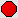and then clicking.
continued on the next slide...

# Exercise - jGRASP Debugger

• Now you can debug the program by clicking on the debug icon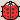(looks like a ladybug).
• The debugger will now pause every time we reach line 5.
• You can see the values of `i` and `number` in the variables tab (should be on the left side of the screen).
• To continue running, press the top-left play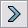or step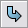buttons.
• continued on the next slide...

# JGrasp Debugger

• Using this approach, fill in the table below indicating what value `number` has when `i` has the given value. Keep in mind that you are figuring out what value `number` has just before it executes line 5. The line of code with the --> pointing to it and the ---- box around it is the line that the debugger is about to execute.

 `i = 1, number = ` `42` `i = 2, number = ` `9` `i = 3, number = ` `24` `i = 4, number = ` `64`

continued on the next slide...

# Exercise - jGRASP Debugger

• Click on the stop-signa second time to get rid of it and then set a new stop point on line number 7. This will allow you to find out the value of `number` after the loop is done executing.
• Again press "play"to start the programming running again.
• At line 7, what is the value of `number`? 69

# Graphics

Now we'll explore several exercises related to drawing graphics. (none)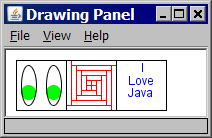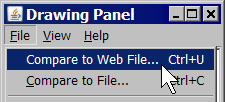• We'll use a provided class `DrawingPanel` that works with Java classes `Graphics` (a "pen" for drawing) and `Color`.
• Download `DrawingPanel.java` by right-clicking the link and selecting "save as".
• You can't open DrawingPanel by clicking on an icon. But if you make a DrawingPanel in Java (which we'll do soon!), a panel will open in a pop-up! You can interact with this window with your mouse, and can check the correctness of your drawings in `DrawingPanel` by clicking File, Compare to Web File....

# Exercise : Graphics

DrawingPanel is like a canvas on which you can draw, and Graphics is like a paint brush.

The coordinate system for the DrawingPanel is different from the one you might be used to. Y coordinates get more positive the further down they are, and more negative the further up they are.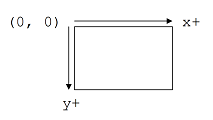# Exercise - Continued

Here is a small segment of code that uses the DrawingPanel and Graphics to start a new canvas to draw on.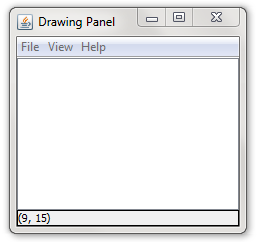``` 1 2 3 4 5 6 7 8 9 10 11``` ```import java.awt.*; public class Draw { public static void main(String[] args) { // Makes a new canvas that is 220px by 150px DrawingPanel panel = new DrawingPanel(220, 150); // This gets the paint brush Graphics g = panel.getGraphics(); } } ```

Copy and paste this code into jGRASP, then save it and compile it. You should store this program in the same directory where you saved `DrawingPanel.java`.

# Exercise : Graphics 2

You can draw shapes on the canvas using your Graphics paint brush. Here are some things you can ask your paint brush to draw:

• `g.drawLine(x1, y1, x2, y2);`
This will draw a line from (x1, y1) to (x2, y2)
• `g.drawOval(x, y, width, height);`
This will draw the biggest oval that fits within the box of size width * height with the top left corner at (x, y).
• `g.drawRect(x, y, width, height);`
This will draw a rectangle of size width x height with the top left at (x, y)

# Exercise - Continued

Try drawing the following on a 500 by 500 canvas: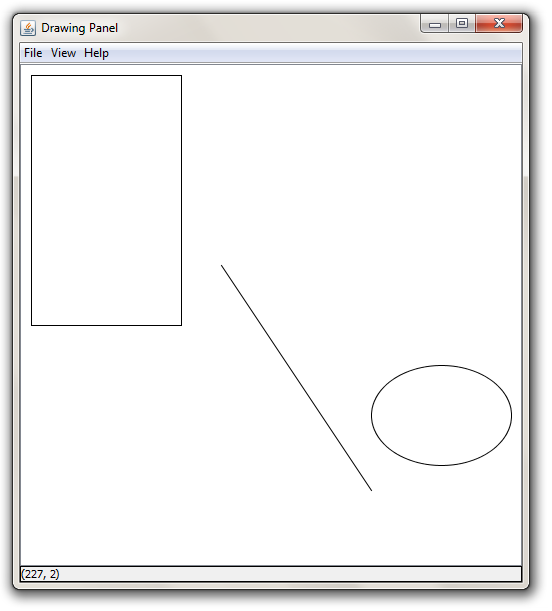• A line starting at (200, 200) and going to (350, 425)
• A rectangle starting at (10, 10) with a width of 150 and a height of 250.
• An oval starting at (350, 300) with a width of 140 and a height of 100.

# Exercise - Solution

 ``` 1 2 3 4 5 6 7 8 9 10 11 12``` ```import java.awt.*; public class Draw { public static void main(String[] args) { DrawingPanel panel = new DrawingPanel(500, 500); Graphics g = panel.getGraphics(); g.drawLine(200, 200, 350, 425); g.drawRect(10, 10, 150, 250); g.drawOval(350, 300, 140, 100); } } ```

# Exercise : Graphics 3

You can also draw shapes that are filled in, instead of just an outline:

• `g.fillOval(x, y, width, height);`
• `g.fillRect(x, y, width, height);`

# Exercise - Continued

Try drawing the following on a 300 by 300 canvas: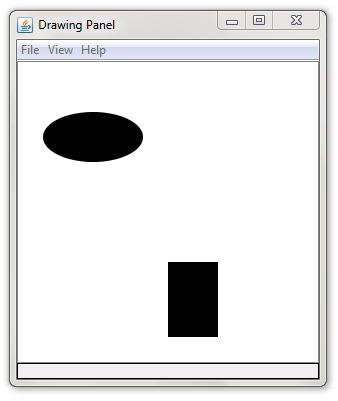• A filled rectangle starting at (150, 200) with a width of 50 and a height of 75.
• A filled oval starting at (25, 50) with a width of 100 and a height of 50.

# Exercise - Solution

 ``` 1 2 3 4 5 6 7 8 9 10 11``` ```import java.awt.*; public class Draw { public static void main(String[] args) { DrawingPanel panel = new DrawingPanel(300, 300); Graphics g = panel.getGraphics(); g.fillRect(150, 200, 50, 75); g.fillOval(25, 50, 100, 50); } } ```

# Exercise : Graphics 4

Here is a list of some colors:

• Color.RED
• Color.BLUE
• Color.BLACK
• Color.YELLOW
• Color.ORANGE
• Color.GREEN
• Color.LIGHT_GRAY
• Color.MAGENTA

You can also change the color of what you draw. It helps to think about changing colors as dipping your paint brush (Graphics) into a can of paint.

• `g.setColor(Color.RED);`
This will change the color of your paint brush.
• `panel.setBackground(Color.BLUE);`
This will change the color of the canvas.

# Exercise - Continued

Try drawing the following on a 300 by 300 canvas that is light gray in color: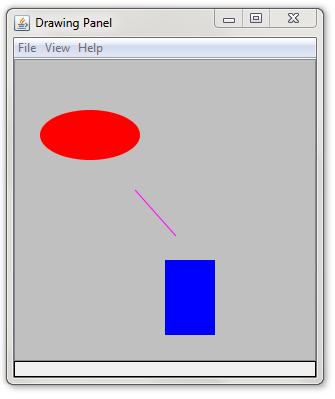• A blue filled rectangle starting at (150, 200) with a width of 50 and a height of 75.
• A red filled oval starting at (25, 50) with a width of 100 and a height of 50.
• A magenta line starting at (120, 130) going to (160, 175)

# Exercise - Solution

 ``` 1 2 3 4 5 6 7 8 9 10 11 12 13 14 15``` ```import java.awt.*; public class Draw { public static void main(String[] args) { DrawingPanel panel = new DrawingPanel(300, 300); Graphics g = panel.getGraphics(); panel.setBackground(Color.LIGHT_GRAY); g.setColor(Color.BLUE); g.fillRect(150, 200, 50, 75); g.setColor(Color.RED); g.fillOval(25, 50, 100, 50); g.setColor(Color.MAGENTA); g.drawLine(120, 130, 160, 175); } } ```

# Exercise : Face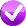Write a complete Java program that draws the following output: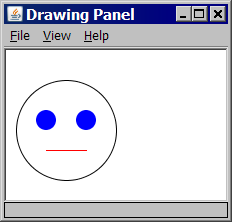• window size: 220 x 150 px
• overall face circle: 100 px diameter; top-left corner at (10, 30)
• eyes: blue circles, 20 px diameter; top-left at (30, 60) and (70, 60)
• mouth: red line from (40, 100) to (80, 100)

 ``` 1 2 3 4 5 6 7 8 9 10 11 12 13 14 15``` ```import java.awt.*; public class Face1 { public static void main(String[] args) { DrawingPanel panel = new DrawingPanel(220, 150); Graphics g = panel.getGraphics(); g.drawOval(10, 30, 100, 100); // face outline g.setColor(Color.BLUE); g.fillOval(30, 60, 20, 20); // eyes g.fillOval(70, 60, 20, 20); g.setColor(Color.RED); // mouth g.drawLine(40, 100, 80, 100); } } ```

# Exercise : Parameter Mystery

(Review this type of problem in lab 3!) Fill in the boxes with the output that each method call produces:

```public static void main(String[] args) {
String song = "good";
String good = "hear";
String walk = "talk";
String talk = "feel";
String feel = "walk";
claim(feel, song, feel);       // to walk the walk is good
claim(good, hear, song);       // to hear the good is bad
claim(talk, "song", feel);     // to feel the walk is song
claim("claim", talk, walk);    // to claim the talk is feel
}
public static void claim(String hear, String good, String song) {
System.out.println("to " + hear + " the " + song + " is " + good);
}
```

# Checkpoint: Congratulations!

Nice job making it this far--labs are tough! Feel free to work with the person next to you for the remaining slides. Labs are a unique opportunity (unlike homework) to collaborate directly on ideas, and practice peer programming.

These next problems get a little more challenging as we explore earlier concepts further.

We put a lot of problems in here so that you have plenty to refer back to later when working on homework. Don't feel bad if you don't finish all of them--Brett can't finish them all in a 50 minute lab, either! :)Forest the cat says good job!

# Exercise : Syntax errors

• The following Java program has several errors. Can you find all of them?  ``` 1 2 3 4 5 6 7 8 9 10 11 12 13 14 15 16 ``` ```public class Parameters { public static void main() { double bubble = 867.5309; double x = 10.01; printer(double x, double y); printer(x); printer("barack", "obama"); System.out.println("z = " + z); } public static void printer(x, y double) { int z = 5; System.out.println("x = " + double x + " and y = " + y); System.out.println("The value from main is: " + bubble); } } ```
• Copy and paste the code into jGrasp and see if you can fix the errors.

# Exercise - Corrected version

Here is a corrected version of the program:
 ``` 1 2 3 4 5 6 7 8 9 10 11 12 13 14 15 16 17 18``` ```public class Parameters { public static void main(String[] args) { double bubble = 867.5309; double x = 10.01; double y = 5.3; // need to declare + initialize var y before we can use it printer(double x, double y); // don't put variable types in method calls! printer(x, 0.0); printer("barack", "obama"); // incorrect types of parameters passed int z = 5; System.out.println("z = " + z); } public static void printer(double x, y double double y) { int z = 5; System.out.println("x = " + double x + " and y = " + y); System.out.println("The value from main is: " + bubble); } } ```

# Exercise : Syntax errors

• The following program contains mistakes! What are they? Once you think you've found them all, compile/run your corrected code.  ``` 1 2 3 4 5 6 7 8 9 10 11 12 13 14 ``` ```import Java.*; public class PrettyPicture { public static void main(String[] args) { DrawingPanel panel = DrawingPanel(220, 150); setBackgroundColor(Color.YELLOW); Graphics g = panel.Graphics(); panel.setColor(new Color.BLUE); g.drawRectangle(50, 25); g.setColor("RED"); g.fillEllipse(130, 25, 42.1, 40.5); } } ```

# Exercise - corrected version

• Here is a corrected version of the program:  ``` 1 2 3 4 5 6 7 8 9 10 11 12 13 14``` ```import java.awt.*; public class PrettyPicture { public static void main(String[] args) { DrawingPanel panel = new DrawingPanel(220, 150); panel.setBackgroundColor(Color.YELLOW); Graphics g = panel.getGraphics(); g.setColor(new Color.BLUE); g.drawRectangle(50, 25, 10, 10); g.setColor(Color.RED); g.fillOval(130, 25, 42, 40); // parameters for this method must be integers } } ```

# Exercise : Parameter MysteryFill in the boxes with the output that each method call would produce:
```public static void main(String[] args) {
String one = "two";
String two = "three";
String three = "1";
int number = 20;

sentence(one, two, 3);                  // three times two = 6
sentence(two, three, 14);               // 1 times three = 28
sentence(three, three, number + 1);     // 1 times 1 = 42
sentence(three, two, 1);                // three times 1 = 2
sentence("eight", three, number / 2);   // 1 times eight = 20
}

public static void sentence(String three, String one, int number) {
System.out.println(one + " times " + three + " = " + (number * 2));
}

```

# Exercise : Printing `String`s• Write a method called `printStrings` that accepts a `String` and a number of repetitions as parameters and prints that `String` the given number of times. For example, the call:

```printStrings("abc", 5);
```

will print the following output:

```abcabcabcabcabc
```
• Complete the program by creating a full Java class with a `main` method. Try calling your method several times from `main` and see what happens when you pass different values.
• Check your work on PracticeIt by clicking the purple checkmark at the top of the page, and copy/pasting your `printStrings` method (not your full code)!

# Exercise : Stairs loop table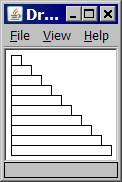Consider the output at right. The first stair's top-left corner is at position (5, 5). The first stair is 10x10 px in size. Each stair is 10px wider than the one above it.

Fill in the table below with the coordinates and sizes of the first five stairs. Note which values change and which ones stay the same.

stair x y width height
`1`
`5`
`5`
`10`
`10`
`2`
`5`
`15`
`20`
`10`
`3`
`5`
`25`
`30`
`10`
`4`
`5`
`35`
`40`
`10`
`5`
`5`
`45`
`50`
`10`

# Exercise : StairsWrite a complete Java program to draw the stairs. Copy/paste the code template below into jGRASP and fill in your own expressions or values for each stair's x, y, width, and height.

Use your table from the previous slide to help you find the correct expressions. The values that change for each stair should become expressions in terms of the loop counter variable, `i`.

 ``` 1 2 3 4 5 6 7 8 9 10 11``` ```import java.awt.*; public class Stairs1 { public static void main(String[] args) { DrawingPanel panel = new DrawingPanel(110, 110); Graphics g = panel.getGraphics(); for (int i = 0; i < 10; i++) { g.drawRect(x, y, width, height); } } } ```

# Exercise : Stairs 2Modify your stairs program to draw one (or all) of the following outputs. Modify only the body in your `for` loop. (You may want to make a new table to find the expressions for x, y, width, and height.)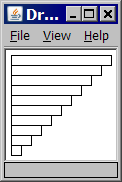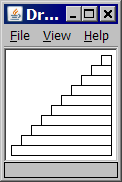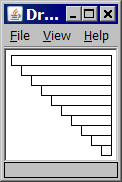To get each output, change the `for` loop body to the following:

```// output 2
g.drawRect(5, 5 + 10*i, 100 - 10*i, 10);
```
```// output 3
g.drawRect(95 - 10*i, 5 + 10*i, 10 + 10*i, 10);
```
```// output 4
g.drawRect(5 + 10*i, 5 + 10*i, 100 - 10*i, 10);
```

# Parameterized methods and Graphics

When you want to divide a graphical program into multiple drawing methods, you must pass Graphics g as a parameter in addition to any other parameters. Example:

 ``` 1 2 3 4 5 6 7 8 9 10 11``` ```public static void main(String[] args) { DrawingPanel panel = new DrawingPanel(400, 300); Graphics g = panel.getGraphics(); ... drawStuff(g, 13, 52, 7); } public static void drawStuff(Graphics g, int a, int b, int c) { g.drawLine(a, 45, b, c); ... } ```

# Exercise : Face 1+2Suppose you have an existing program that draws the "face" figure at right. Let's modify the program using methods and parameters so that we can draw several faces at different locations.

continued on the next slide...

# Exercise : Face 2Modify the `Face` program to draw the following output. Write a parameterized method that draws a face at different positions.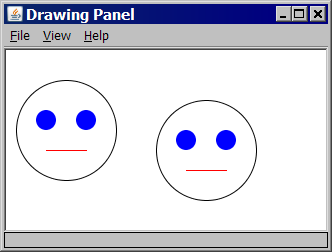• window size: 320 x 180 px
• faces' top-left corners at (10, 30) and (150, 50)

 ``` 1 2 3 4 5 6 7 8 9 10 11 12 13 14 15 16 17 18 19``` ```import java.awt.*; public class Face2 { public static void main(String[] args) { DrawingPanel panel = new DrawingPanel(320, 180); Graphics g = panel.getGraphics(); drawFace(g, 10, 30); drawFace(g, 150, 50); } public static void drawFace(Graphics g, int x, int y) { g.setColor(Color.BLACK); g.drawOval(x, y, 100, 100); g.setColor(Color.BLUE); g.fillOval(x + 20, y + 30, 20, 20); g.fillOval(x + 60, y + 30, 20, 20); g.setColor(Color.RED); g.drawLine(x + 30, y + 70, x + 70, y + 70); } } ```

# Exercise : Face 3Modify your previous Java program to draw the following output. Use a `for` loop with your parameterized method to draw faces at different positions.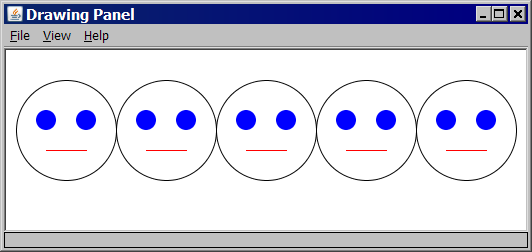• window size: 520 x 180 px
• faces' top-left at (10, 30), (110, 30), (210, 30), (310, 30), and (410, 30)

 ``` 1 2 3 4 5 6 7 8 9 10 11 12 13 14 15 16 17 18 19``` ```import java.awt.*; public class Face3 { public static void main(String[] args) { DrawingPanel panel = new DrawingPanel(520, 180); Graphics g = panel.getGraphics(); for (int i = 0; i < 5; i++) { drawFace(g, 10 + i * 100, 30); } } public static void drawFace(Graphics g, int x, int y) { g.setColor(Color.BLACK); g.drawOval(x, y, 100, 100); g.setColor(Color.BLUE); g.fillOval(x + 20, y + 30, 20, 20); g.fillOval(x + 60, y + 30, 20, 20); g.setColor(Color.RED); g.drawLine(x + 30, y + 70, x + 70, y + 70); } } ```

# Exercise : Parameter MysteryFill in the boxes with the output that each method call would produce:
```public static void main(String[] args) {
String soda = "coke";
String pop = "pepsi";
String coke = "pop";
String pepsi = "soda";
String say = pop;

carbonated(soda, pop, pepsi);        // say pepsi not soda or coke
carbonated(coke, soda, pop);         // say coke not pepsi or pop
carbonated(pop, pepsi, pepsi);       // say soda not soda or pepsi
carbonated("pop", pop, "koolaid");   // say pepsi not koolaid or pop
carbonated(say, "say", pop);         // say say not pepsi or pepsi
}
public static void carbonated(String coke, String soda, String pop) {
System.out.println("say " + soda + " not " + pop + " or " + coke);
}
```

# If you finish them all...

If you finish all the exercises, try out our Practice-It web tool. It lets you solve Java problems from our Building Java Programs textbook.

You can view an exercise, type a solution, and submit it to see if you have solved it correctly.

Choose some problems from the book and try to solve them!